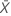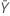# Methods and formulas for Test mean - reference mean for Equivalence Test with Paired Data

The following methods and formulas are used for testing the difference between the test mean and the reference mean.

## Difference (D)

### Notation

TermDescription
DDifferenceTest meanReference mean

## SE of the difference

Minitab uses the following formula to calculate the standard error (SE) of the difference: where S is the standard deviation of the differences as defined below.

### Notation

TermDescription
SEStandard error of the difference
SStandard deviation of the differences
nNumber of pairs of observations
diPairwise differences (X i - Yi), i = 1, ..., nAverage of the pairwise differences

## Equivalence limits

Let k1 be the value that you specify for the lower limit and k2 be the value that you specify for the upper limit. By default, the lower equivalence limit, δ1, is given by:and the upper equivalence limit, δ2, is given by:## Degrees of freedom (DF)

### Notation

TermDescription
vDegrees of freedom
nNumber of pairs of observations

## Confidence interval

### 100(1-α)% CI

By default, Minitab uses the following formula to calculate the 100(1 – α)% confidence interval (CI) for equivalence:

CI = [min(C, Dl), max(C, Du)]

where:

### 100(1-2α)% CI

If you select the option to use the 100(1 – 2α)% CI, then the CI is given by the following formula:

CI = [Dl, Du]

### One-sided intervals

For a hypotheses of Test mean > reference mean or Test mean - reference mean > lower limit, the 100(1 – α)% lower bound is equal to DL.

For a hypothesis of Test mean < reference mean or Test mean - reference mean < upper limit, the 100(1 – α)% upper bound is equal to DU.

### Notation

TermDescription
DDifference between the test mean and the reference mean
SEStandard error
δ1Lower equivalence limit
δ2Upper equivalence limit
vDegrees of freedom
αThe significance level for the test (alpha)
t1-α, vUpper 1 – α critical value for a t-distribution with v degrees of freedom

## T-values

Let t1 be the t-value for the hypothesis,, and let t2 be the t-value for the hypothesis,, whereis the difference between the mean of the test population and the mean of the reference population. By default, the t-values are calculated as follows:

For a hypothesis of Test mean > reference mean, δ1 = 0.

For a hypothesis of Test mean < reference mean, δ 2 = 0.

### Notation

TermDescription
DDifference between the sample test mean and the sample reference mean
SEStandard error of the difference
δ1Lower equivalence limit
δ2Upper equivalence limit

## P-values

The probability, PH0, for each null hypothesis (H0) is given by the following:
H0 P-Value### Notation

TermDescriptionUnknown difference between the mean of the test population and the mean of the reference population
δ1Lower equivalence limit
δ2Upper equivalence limit
vDegrees of freedom
Tt-distribution with v degrees of freedom
t1 t-value for the hypothesist2 t-value for the hypothesis###### Note

For information on how the t-values are calculated, see the section on t-values.